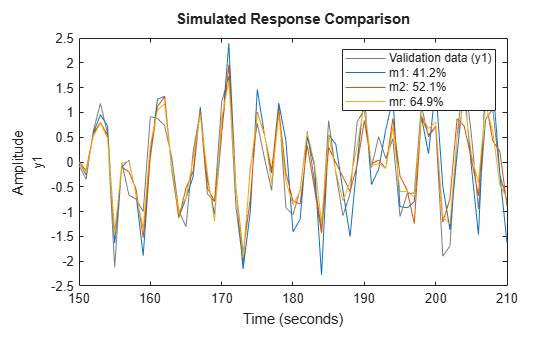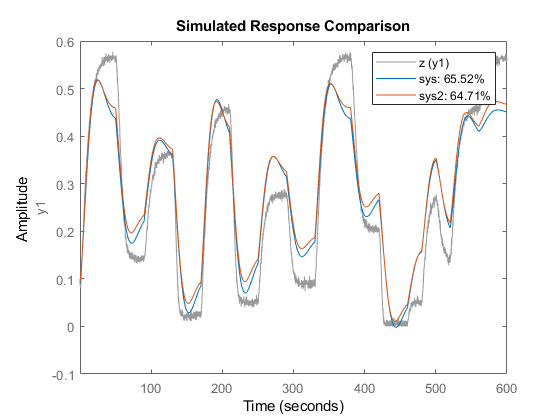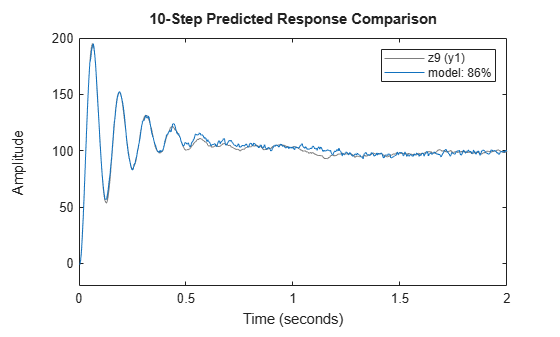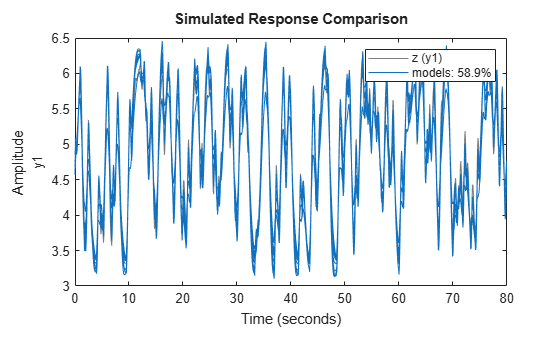# armax

Estimate parameters of ARMAX model using time-domain data

## Syntax

``````sys = armax(data,[na nb nc nk])``````
``````sys = armax(data,[na nb nc nk],Name,Value)``````
``sys = armax(data,init_sys)``
``sys = armax(data,___,opt)``

## Description

example

``````sys = armax(data,[na nb nc nk])``` returns an `idpoly` model, `sys`, with estimated parameters and covariance (parameter uncertainties). Estimates the parameters using the prediction-error method and specified polynomial orders.```

example

``````sys = armax(data,[na nb nc nk],Name,Value)``` returns an `idpoly` model, `sys`, with additional options specified by one or more `Name,Value` pair arguments.```

example

````sys = armax(data,init_sys)` estimates a polynomial model using a discrete-time linear model `init_sys` to configure the initial parameterization.```

example

````sys = armax(data,___,opt)` specifies estimation options using the option set `opt`.```

## Input Arguments

collapse all

Time-domain estimation data, specified as an `iddata` object. You cannot use frequency-domain data for estimating ARMAX models.

Polynomial orders of an ARMAX Model, specified as a 1-by-4 vector, `[na nb nc nk]`.

For a model with Ny outputs and Nu inputs:

• `na` is the order of the polynomial A(q), specified as an Ny-by-Ny matrix of nonnegative integers.

• `nb` is the order of the polynomial B(q) + 1, specified as an Ny-by-Nu matrix of nonnegative integers.

• `nc` is the order of the polynomial C(q), specified as a column vector of nonnegative integers of length Ny.

• `nk` is the input-output delay expressed as fixed leading zeros of the B polynomial.

Specify `nk` as an Ny-by-Nu matrix of nonnegative integers.

System for configuring initial parametrization of `sys`, specified as a discrete-time linear model. You obtain `init_sys` by either performing an estimation using measured data or by direct construction using commands such as `idpoly` and `idss`.

If `init_sys` is an ARMAX model, `armax` uses the parameter values of `init_sys` as the initial guess for estimation. To configure initial guesses and constraints for A(q), B(q), and C(q), use the `Structure` property of `init_sys`. For example:

• To specify an initial guess for the A(q) term of `init_sys`, set `init_sys.Structure.A.Value` as the initial guess.

• To specify constraints for the B(q) term of `init_sys`:

• set `init_sys.Structure.B.Minimum` to the minimum B(q) coefficient values.

• set `init_sys.Structure.B.Maximum` to the maximum B(q) coefficient values.

• set `init_sys.Structure.B.Free` to indicate which B(q) coefficients are free for estimation.

If `init_sys` is not a polynomial model of ARMAX structure, the software first converts `init_sys` to an ARMAX model. `armax` uses the parameters of the resulting model as the initial guess for estimating `sys`.

If `opt` is not specified, and `init_sys` was obtained by estimation, then the estimation options from `init_sys.Report.OptionsUsed` are used.

Estimation options for ARMAX model identification, specified as an `armaxOptions` option set.

### Name-Value Pair Arguments

Specify optional comma-separated pairs of `Name,Value` arguments. `Name` is the argument name and `Value` is the corresponding value. `Name` must appear inside quotes. You can specify several name and value pair arguments in any order as `Name1,Value1,...,NameN,ValueN`.

Input delays, specified as the comma-separated pair consisting of `'InputDelay'` and one of the following:

• `Nu`-by-1 vector, where `Nu` is the number of inputs — Each entry is a numerical value representing the input delay for the corresponding input channel. Specify input delays as integer multiples of the sample time `Ts`. For example, `InputDelay = 3` means a delay of three sampling periods.

• Scalar value — The same delay is applied to all input channels.

Transport delays for each input/output pair, specified as the comma-separated pair consisting of `'IODelay'` and one of the following:

• `Ny`-by-`Nu` matrix, where `Ny` is the number of outputs and `Nu` is the number of inputs — Each entry is an integer value representing the transport delay for the corresponding input/output pair. Specify transport delays as integers denoting delay of a multiple of the sample time `Ts`.

• Scalar value — The same delay is applied to all input/output pairs.

`'IODelay'` is useful as a replacement for the `nk` order. You can factor out `max(nk-1,0)` lags as the `IODelay` value. For `nk`>1, `armax(na,nb,nk)` is equivalent to `armax(na,nb,1,'IODelay',nk-1)`.

Addition of integrators in noise channel, specified as the comma-separated pair consisting of `'IntegrateNoise'` and a logical vector of length Ny, where Ny is the number of outputs.

Setting `IntegrateNoise` to `true` for a particular output results in the model:

`$A\left(q\right)y\left(t\right)=B\left(q\right)u\left(t-nk\right)+\frac{C\left(q\right)}{1-{q}^{-1}}e\left(t\right)$`

Where, $\frac{1}{1-{q}^{-1}}$ is the integrator in the noise channel, e(t).

Use `IntegrateNoise` to create ARIMA or ARIMAX models.

For example,

```load iddata9 z9; z9.y = cumsum(z9.y); % integrated data sys = armax(z9,[4 1],'IntegrateNoise',true); compare(z9,sys,10) % 10-step ahead prediction```

## Output Arguments

collapse all

ARMAX model that fits the given estimation data, returned as a discrete-time `idpoly` object. This model is created using the specified model orders, delays, and estimation options.

Information about the estimation results and options used is stored in the `Report` property of the model. `Report` has the following fields:

Report FieldDescription
`Status`

Summary of the model status, which indicates whether the model was created by construction or obtained by estimation.

`Method`

Estimation command used.

`InitialCondition`

Handling of initial conditions during model estimation, returned as one of the following values:

• `'zero'` — The initial conditions were set to zero.

• `'estimate'` — The initial conditions were treated as independent estimation parameters.

• `'backcast'` — The initial conditions were estimated using the best least squares fit.

This field is especially useful to view how the initial conditions were handled when the `InitialCondition` option in the estimation option set is `'auto'`.

`Fit`

Quantitative assessment of the estimation, returned as a structure. See Loss Function and Model Quality Metrics for more information on these quality metrics. The structure has the following fields:

FieldDescription
`FitPercent`

Normalized root mean squared error (NRMSE) measure of how well the response of the model fits the estimation data, expressed as a percentage.

`LossFcn`

Value of the loss function when the estimation completes.

`MSE`

Mean squared error (MSE) measure of how well the response of the model fits the estimation data.

`FPE`

Final prediction error for the model.

`AIC`

Raw Akaike Information Criteria (AIC) measure of model quality.

`AICc`

Small sample-size corrected AIC.

`nAIC`

Normalized AIC.

`BIC`

Bayesian Information Criteria (BIC).

`Parameters`

Estimated values of model parameters.

`OptionsUsed`

Option set used for estimation. If no custom options were configured, this is a set of default options. See `armaxOptions` for more information.

`RandState`

State of the random number stream at the start of estimation. Empty, `[]`, if randomization was not used during estimation. For more information, see `rng` in the MATLAB® documentation.

`DataUsed`

Attributes of the data used for estimation, returned as a structure with the following fields:

FieldDescription
`Name`

Name of the data set.

`Type`

Data type.

`Length`

Number of data samples.

`Ts`

Sample time.

`InterSample`

Input intersample behavior, returned as one of the following values:

• `'zoh'` — Zero-order hold maintains a piecewise-constant input signal between samples.

• `'foh'` — First-order hold maintains a piecewise-linear input signal between samples.

• `'bl'` — Band-limited behavior specifies that the continuous-time input signal has zero power above the Nyquist frequency.

`InputOffset`

Offset removed from time-domain input data during estimation. For nonlinear models, it is `[]`.

`OutputOffset`

Offset removed from time-domain output data during estimation. For nonlinear models, it is `[]`.

`Termination`

Termination conditions for the iterative search used for prediction error minimization. Structure with the following fields:

FieldDescription
`WhyStop`

Reason for terminating the numerical search.

`Iterations`

Number of search iterations performed by the estimation algorithm.

`FirstOrderOptimality`

$\infty$-norm of the gradient search vector when the search algorithm terminates.

`FcnCount`

Number of times the objective function was called.

`UpdateNorm`

Norm of the gradient search vector in the last iteration. Omitted when the search method is `'lsqnonlin'` or `'fmincon'`.

`LastImprovement`

Criterion improvement in the last iteration, expressed as a percentage. Omitted when the search method is `'lsqnonlin'` or `'fmincon'`.

`Algorithm`

Algorithm used by `'lsqnonlin'` or `'fmincon'` search method. Omitted when other search methods are used.

For estimation methods that do not require numerical search optimization, the `Termination` field is omitted.

For more information on using `Report`, see Estimation Report.

## Examples

collapse all

Estimate a regularized ARMAX model by converting a regularized ARX model.

`load regularizationExampleData.mat m0simdata;`

Estimate an unregularized ARMAX model of order 15.

`m1 = armax(m0simdata(1:150),[30 30 30 1]);`

Estimate a regularized ARMAX model by determining Lambda value by trial and error.

```opt = armaxOptions; opt.Regularization.Lambda = 1; m2 = armax(m0simdata(1:150),[30 30 30 1],opt);```

Obtain a lower-order ARMAX model by converting a regularized ARX model followed by order reduction.

```opt1 = arxOptions; [L,R] = arxRegul(m0simdata(1:150),[30 30 1]); opt1.Regularization.Lambda = L; opt1.Regularization.R = R; m0 = arx(m0simdata(1:150),[30 30 1],opt1); mr = idpoly(balred(idss(m0),7));```

Compare the model outputs against data.

```opt2 = compareOptions('InitialCondition','z'); compare(m0simdata(150:end),m1,m2,mr,opt2);```Estimate an ARMAX model from measured data and specify the estimation options.

Estimate an ARMAX model with simulation focus, using `'lm'` as the search method and maximum number of search iterations set to 10.

```load twotankdata; z = iddata(y,u,0.2); opt = armaxOptions; opt.Focus = 'simulation'; opt.SearchMethod = 'lm'; opt.SearchOptions.MaxIterations = 10; opt.Display = 'on'; sys = armax(z,[2 2 2 1],opt);```

The termination conditions for measured component of the model shown in the progress viewer is that the maximum number of iterations were reached.

To improve results, re-estimate the model using a greater value for `MaxIterations`, or continue iterations on the previously estimated model as follows:

```sys2 = armax(z,sys); compare(z,sys,sys2)```where `sys2` refines the parameters of `sys` to improve the fit to data.

Estimate a 4th order ARIMA model for univariate time-series data.

```load iddata9; z9.y = cumsum(z9.y); % integrated data model = armax(z9,[4 1],'IntegrateNoise',true); compare(z9,model,10) % 10-step ahead prediction```Estimate ARMAX models of varying orders iteratively from measured data.

Estimate ARMAX models of orders varying between 1 and 4 for dryer data.

```load dryer2; z = iddata(y2,u2,0.08,'Tstart',0); na = 2:4; nc = 1:2; nk = 0:2; models = cell(1,18); ct = 1; for i = 1:3 na_ = na(i); nb_ = na_; for j = 1:2 nc_ = nc(j); for k = 1:3 nk_ = nk(k); models{ct} = armax(z,[na_ nb_ nc_ nk_]); ct = ct+1; end end end```

Stack the estimated models and compare their simulated responses to estimation data `z`.

```models = stack(1,models{:}); compare(z,models)````load iddata2 z2`

Estimate a state-space model of order 3 from the estimation data.

`sys0 = n4sid(z2,3);`

Estimate an ARMAX model using the previously estimated state-space model as an initial guess.

`sys = armax(z2,sys0);`

collapse all

### ARMAX Model

The ARMAX model structure is:

A more compact way to write the difference equation is:

`$A\left(q\right)y\left(t\right)=B\left(q\right)u\left(t-{n}_{k}\right)+C\left(q\right)e\left(t\right)$`

where,

• $y\left(t\right)$— Output at time $t$.

• ${n}_{a}$ — Number of poles.

• ${n}_{b}$ — Number of zeroes plus 1.

• ${n}_{c}$ — Number of C coefficients.

• ${n}_{k}$ — Number of input samples that occur before the input affects the output, also called the dead time in the system.

• $y\left(t-1\right)\dots y\left(t-{n}_{a}\right)$ — Previous outputs on which the current output depends.

• $u\left(t-{n}_{k}\right)\dots u\left(t-{n}_{k}-{n}_{b}+1\right)$ — Previous and delayed inputs on which the current output depends.

• $e\left(t-1\right)\dots e\left(t-{n}_{c}\right)$ — White-noise disturbance value.

The parameters `na`, `nb`, and `nc` are the orders of the ARMAX model, and `nk` is the delay. q is the delay operator. Specifically,

`$A\left(q\right)=1+{a}_{1}{q}^{-1}+\dots +{a}_{{n}_{a}}{q}^{-{n}_{a}}$`
`$B\left(q\right)={b}_{1}+{b}_{2}{q}^{-1}+\dots +{b}_{{n}_{b}}{q}^{-{n}_{b}+1}$`
`$C\left(q\right)=1+{c}_{1}{q}^{-1}+\dots +{c}_{{n}_{c}}{q}^{-{n}_{c}}$`

If `data` is a time series that has no input channels and one output channel, then `armax` calculates an ARMA model for the time series

`$A\left(q\right)y\left(t\right)=C\left(q\right)e\left(t\right)$`

In this case,

```orders = [na nc] ```

### ARIMAX Model

An ARIMAX model structure is similar to ARMAX, except that it contains an integrator in the noise source e(t):

`$A\left(q\right)y\left(t\right)=B\left(q\right)u\left(t-nk\right)+\frac{C\left(q\right)}{\left(1-{q}^{-1}\right)}e\left(t\right)$`

If there are no inputs, the structure reduces to an ARIMA model:

`$A\left(q\right)y\left(t\right)=\frac{C\left(q\right)}{\left(1-{q}^{-1}\right)}e\left(t\right)$`

## Tips

• Use the `IntegrateNoise` property to add integrators to the noise source.

## Algorithms

An iterative search algorithm minimizes a robustified quadratic prediction error criterion. The iterations are terminated when any of the following is true:

• Maximum number of iterations is reached.

• Expected improvement is less than the specified tolerance.

• Lower value of the criterion cannot be found.

You can get information about the stopping criteria using `sys.Report.Termination`.

Use the `armaxOptions` option set to create and configure options affecting the estimation results. In particular, set the search algorithm attributes, such as `MaxIterations` and `Tolerance`, using the `'SearchOptions'` property.

When you do not specify initial parameter values for the iterative search as an initial model, they are constructed in a special four-stage LS-IV algorithm.

The cutoff value for the robustification is based on the `Advanced.ErrorThreshold` estimation option and on the estimated standard deviation of the residuals from the initial parameter estimate. It is not recalculated during the minimization. By default, no robustification is performed; the default value of `ErrorThreshold` option is 0.

To ensure that only models corresponding to stable predictors are tested, the algorithm performs a stability test of the predictor. Generally, both $C\left(q\right)$ and $F\left(q\right)$ (if applicable) must have all zeros inside the unit circle.

Minimization information is displayed on the screen when the estimation option `'Display'` is `'On'` or `'Full'`. With `'Display'` `='Full'`, both the current and the previous parameter estimates are displayed in column-vector form, listing parameters in alphabetical order. Also, the values of the criterion function (cost) are given and the Gauss-Newton vector and its norm are also displayed. With ```'Display' = 'On'```, only the criterion values are displayed.

## Alternatives

`armax` does not support continuous-time model estimation. Use `tfest` to estimate a continuous-time transfer function model, or `ssest` to estimate a continuous-time state-space model.

`armax` supports only time-domain data. For frequency-domain data, use `oe` to estimate an Output-Error (OE) model.

## References

Ljung, L. System Identification: Theory for the User, Upper Saddle River, NJ: Prentice-Hall PTR, 1999. See chapter about computing the estimate.

## Extended Capabilities